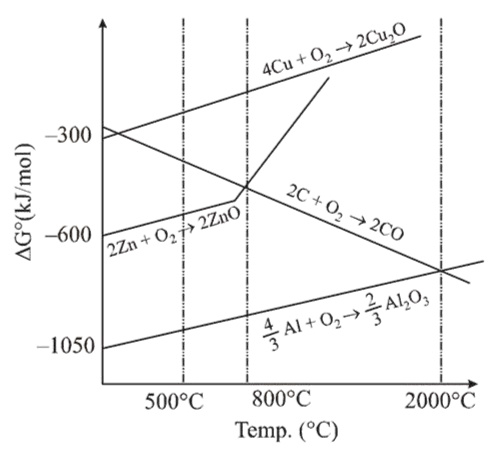# The correct statement regarding the given Ellingham diagram is:Question:

The correct statement regarding the given Ellingham diagram is:1. At $1400^{\circ} \mathrm{C}, \mathrm{Al}$ can be used for the extraction of $\mathrm{Zn}$ from $\mathrm{ZnO}$

2. At $500^{\circ} \mathrm{C}$, coke can be used for the extraction of $\mathrm{Zn}$ from $\mathrm{ZnO}$

3. Coke cannot be used for the extraction of $\mathrm{Cu}$ from $\mathrm{Cu}_{2} \mathrm{O}$.

4. At $800^{\circ} \mathrm{C}, \mathrm{Cu}$ can be used for the extraction of $\mathrm{Zn}$ from $\mathrm{ZnO}$

Correct Option: 1

Solution:

In the given Ellingham diagram, the metal which has a lower value of $\Delta G^{\circ}$ (more negative) can reduce a metal oxide whose curve lies above it. So, Al can reduce $\mathrm{ZnO}$ at $1400^{\circ} \mathrm{C}$.